# Pyspark – Filter dataframe based on multiple conditions

• Last Updated : 04 Jul, 2021

In this article, we are going to see how to Filter dataframe based on multiple conditions.

Let’s Create a Dataframe for demonstration:

## Python3

 `# importing module``import` `pyspark`` ` `# importing sparksession from pyspark.sql module``from` `pyspark.sql ``import` `SparkSession`` ` `# creating sparksession and giving an app name``spark ``=` `SparkSession.builder.appName(``'sparkdf'``).getOrCreate()`` ` `# list  of students  data``data ``=` `[[``"1"``, ``"Amit"``, ``"DU"``],``        ``[``"2"``, ``"Mohit"``, ``"DU"``],``        ``[``"3"``, ``"rohith"``, ``"BHU"``],``        ``[``"4"``, ``"sridevi"``, ``"LPU"``],``        ``[``"1"``, ``"sravan"``, ``"KLMP"``],``        ``[``"5"``, ``"gnanesh"``, ``"IIT"``]]`` ` `# specify column names``columns ``=` `[``'student_ID'``, ``'student_NAME'``, ``'college'``]`` ` `# creating a dataframe from the lists of data``dataframe ``=` `spark.createDataFrame(data, columns)`` ` `# show dataframe``dataframe.show()`

Output: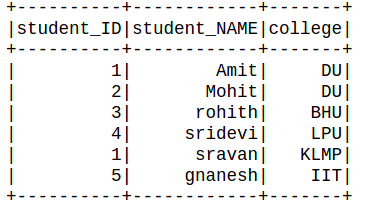### Method 1: Using Filter()

filter(): It is a function which filters the columns/row based on SQL expression or condition.

Syntax: Dataframe.filter(Condition)

Where condition may be given Logcal expression/ sql expression

Example 1: Filter single condition

## Python3

 `dataframe.``filter``(dataframe.college ``=``=` `"DU"``).show()`

Output: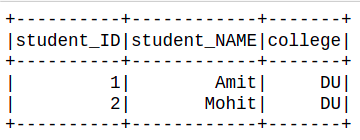Example 2: Filter columns with multiple conditions.

## Python3

 `dataframe.``filter``((dataframe.college ``=``=` `"DU"``) &``                 ``(dataframe.student_ID ``=``=` `"1"``)).show()`

Output: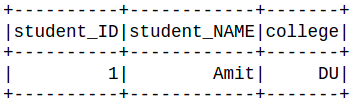### Method 2: Using filter and SQL Col

Here we are going to use the SQL col function, this function refers the column name of the dataframe with dataframe_object.col.

Syntax: Dataframe_obj.col(column_name).

Where, Column_name is refers to the column name of dataframe.

Example 1: Filter column with a single condition.

## Python3

 `# Using SQL col() function``from` `pyspark.sql.functions ``import` `col``dataframe.``filter``(col(``"college"``) ``=``=` `"DU"``).show()`

Output: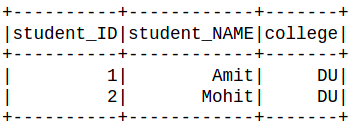Example 2: Filter column with multiple conditions.

## Python3

 `# Using SQL col() function``from` `pyspark.sql.functions ``import` `col`` ` ` ` `dataframe.``filter``((col(``"college"``) ``=``=` `"DU"``) &``                 ``(col(``"student_NAME"``) ``=``=` `"Amit"``)).show()`

Output: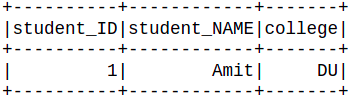### Method 3: Using isin()

isin(): This function takes a list as a parameter and returns the boolean expression. The boolean expression that is evaluated to true if the value of this expression is contained by the evaluated values of the arguments

Syntax: isin(*list)

Where *list is extracted from of list.

Example 1: Filter with a single list.

## Python3

 `list` `=` `[``1``, ``2``]``dataframe.``filter``(dataframe.student_ID.isin(``list``)).show()`

Output: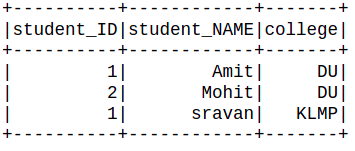Example 2: Filter with multiple lists.

## Python3

 `Id_list ``=` `[``1``, ``2``]``college_list ``=` `[``'DU'``,``'IIT'``]``dataframe.``filter``((dataframe.student_ID.isin(Id_list)) |``                 ``(dataframe.college.isin(college_list))).show()`

Output: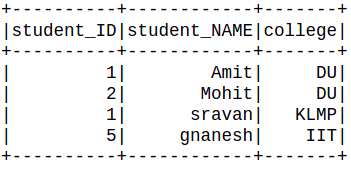### Method 4: Using Startswith and endswith

Here we will use startswith and endswith function of pyspark.

startswith(): This function takes a character as a parameter and searches in the columns string whose string starting with the first character if the condition satisfied then returns True.

Syntax: startswith(character)

Example:

## Python3

 `dataframe.``filter``(dataframe.student_NAME.startswith(``'s'``)).show()`

Output: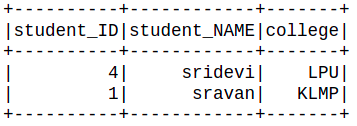endswith(): This function takes a character as a parameter and searches in the columns string whose string ending with the character if the condition satisfied then returns True.

Syntax: endswith(character)

Example:

## Python3

 `dataframe.``filter``(dataframe.student_NAME.endswith(``'t'``)).show()`

Output:Here will use both functions for filtering the dataframe:

## Python3

 `dataframe.``filter``((dataframe.student_NAME.endswith(``'t'``)) &``                 ``(dataframe.student_NAME.startswith(``"A"``))).show()`

Output:My Personal Notes arrow_drop_up